Next: C. Stratified Medium Up: B. Analytical Fourier Transformations Previous: B.2 Rectangular Patterns

# B.3 Numerical Evaluation

In Section 4.4.1 it is shown that aliasing errors are avoided by the analytical transformation of the mask transfer function. Although aliasing is suppressed, the numerical evaluation of the provided analytical formulae (B.6) and (B.8) can introduce errors due to the finite machine precision. Special care has to be taken for si(x) = sin(x)/x since it is an indefinite expression for x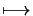0. In (B.6) cancellation errors can occur due to the required subtractions. The following perturbation analysis proves that both formulae (B.6) and (B.8) are numerically robust and stable. Consequently the proposed semi-analytical algorithm for the calculation of the Fourier coefficients of the mask transmission function neither lacks aliasing nor numerical evaluation errors.

The Taylor series expansions of the two functions si(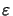) and exp(- j) for ||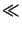1, i.e.,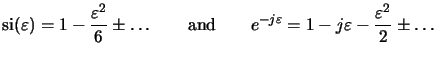(B.7)

readily show that the formula  I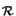(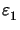/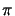,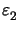/) in (B.8) valid for rectangular patterns is stable for ||,||1 since(B.8)

For triangular patterns the evaluation of the second and third cases in (B.6) is particularly peculiar since cancellation errors are potentially caused by the required subtractions. The two cases are studied separately by inserting the Taylor expansions (B.9) into (B.6) and linearizing the expressions:

• Case II in (B.6):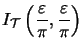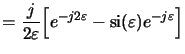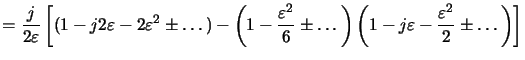(B.9)

• Case III in (B.6):b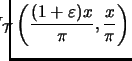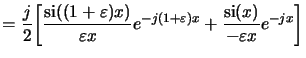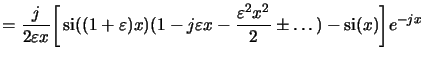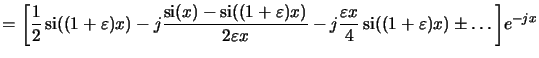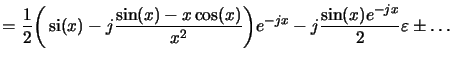(B.10)

Summarizing (B.10) to (B.12),c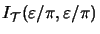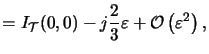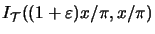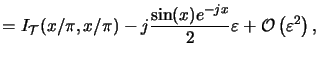(B.11)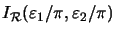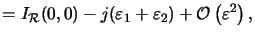proves the robustness of all formulae. In the actual implementation these Taylor expansions are used to avoid numerical instabilities.

#### Footnotes

...):b
Using Taylor expansions for cos(x) = 1 -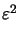x2/2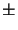... and sin(x) =x... yields: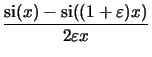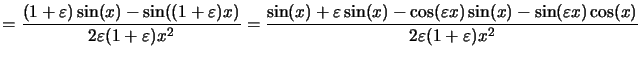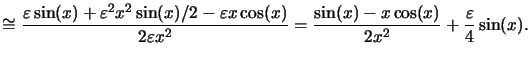...),c
Note that in (B.12) the first term equals the expression for the second case in (B.6) since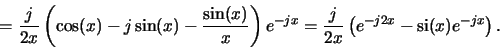Next: C. Stratified Medium Up: B. Analytical Fourier Transformations Previous: B.2 Rectangular Patterns
Heinrich Kirchauer, Institute for Microelectronics, TU Vienna
1998-04-17